Courses

# Test: Data Handling - 1

## 10 Questions MCQ Test Mathematics for Class 5 (V) - CBSE and NCERT Curriculum | Test: Data Handling - 1

Description
This mock test of Test: Data Handling - 1 for Class 5 helps you for every Class 5 entrance exam. This contains 10 Multiple Choice Questions for Class 5 Test: Data Handling - 1 (mcq) to study with solutions a complete question bank. The solved questions answers in this Test: Data Handling - 1 quiz give you a good mix of easy questions and tough questions. Class 5 students definitely take this Test: Data Handling - 1 exercise for a better result in the exam. You can find other Test: Data Handling - 1 extra questions, long questions & short questions for Class 5 on EduRev as well by searching above.
QUESTION: 1

Solution:
QUESTION: 2

Solution:
QUESTION: 3

### Direction: The given bar graph shows the number of residents in 6 cities. Study the graph and answer the question.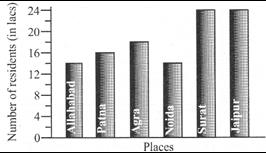Q. How many residents were there altogether in the 6 cities?

Solution:
QUESTION: 4

Direction: The given bar graph shows the number of viewers who watched the 4 channels. Study the graph and answer the question.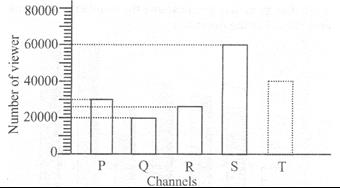Q. How many more viewers watched Channel S than Channel Q?

Solution:
QUESTION: 5

Direction: The given bar graph shows the number of viewers who watched the 4 channels. Study the graph and answer the question.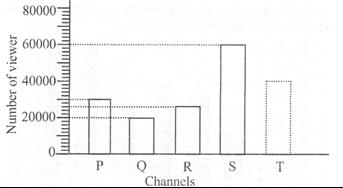Q. There were 150000 viewers altogether. How many viewers watched Channel T?

Solution:
QUESTION: 6

Direction: The Sine graph shows the quantity of petrol Ankit used and the distance his car travelled. Study the graph answer the question.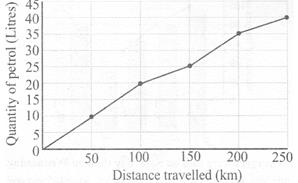Q. How much less petrol was used for 100 km 200 km travelled?

Solution:
QUESTION: 7

Direction: The Sine graph shows the quantity of petrol Ankit used and the distance his car travelled. Study the graph answer the question.What distance was travelled with 20 litres of petrol?

Solution:
QUESTION: 8

Direction: The Sine graph shows the quantity of petrol Ankit used and the distance his car travelled. Study the graph answer the question.Q. What distance was travelled with 35 litres of petrol?

Solution:
QUESTION: 9

Direction: Study the graph and answer the question.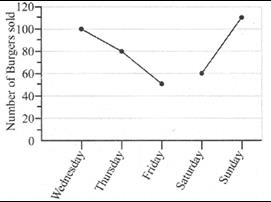Q. The sum of the greatest number of burgers and the smallest number burgers sold is

Solution:
QUESTION: 10

Direction: Study the graph and answer the question.Q. How many less burgers were sold on Saturday than on Wednesday?

Solution: